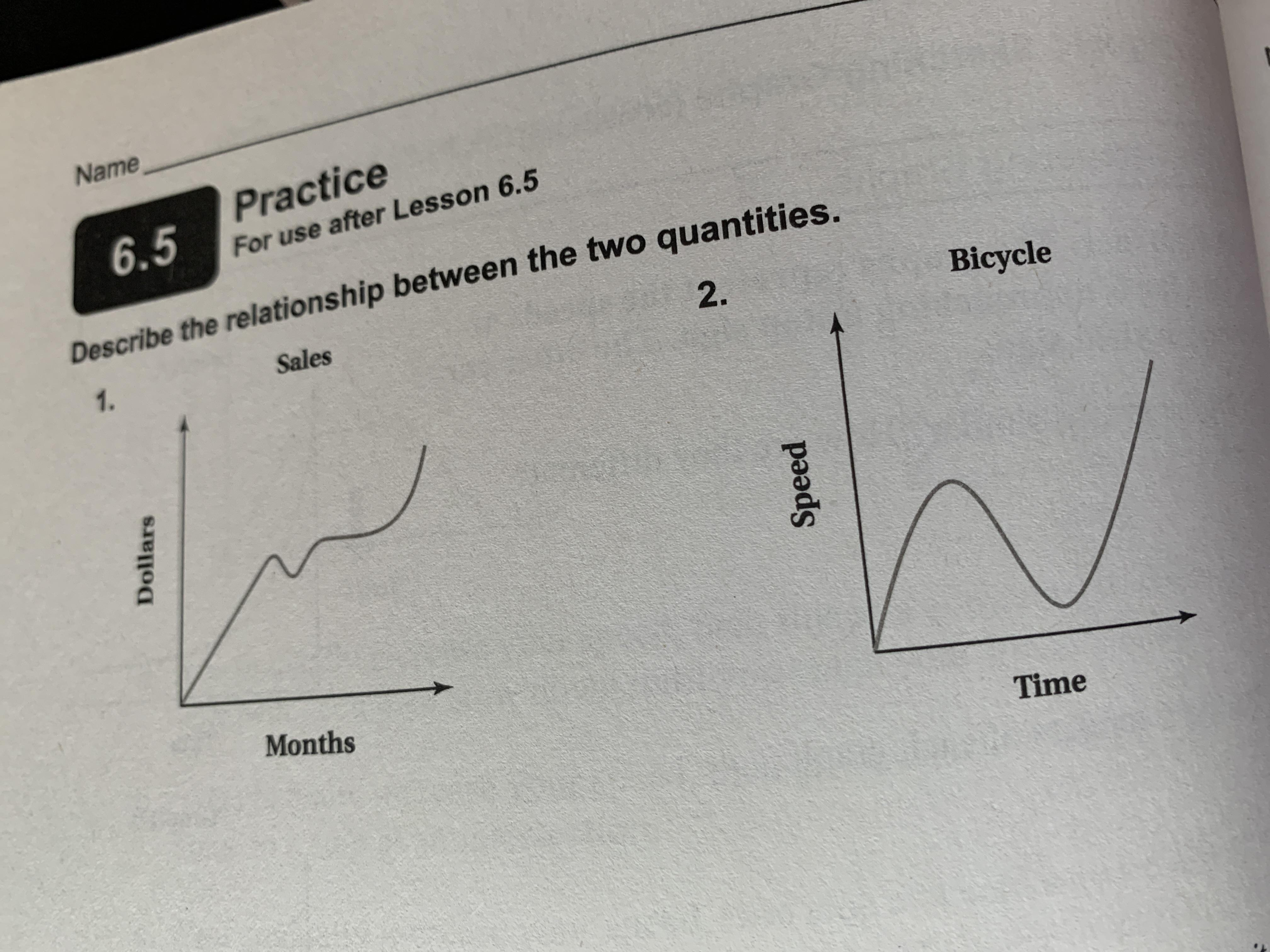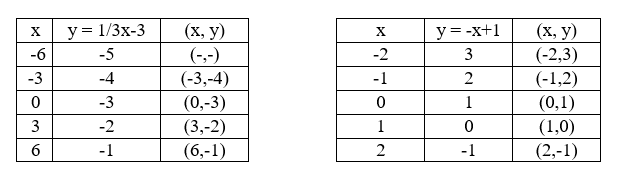Ask question

# Describe the relationship between the two quantities.1 [Graph] 2 [Graph]# Describe the relationship between the two quantities.1 [Graph] 2 [Graph]

Question
Linear equations and graphsasked 2021-03-18

Describe the relationship between the two quantities.## Answers (1)2021-03-19
1.The given graph describes the flow of the sales shown in dollars durring the months.
As can be seen from the graph, the relationship between x (months) and y (dollars) is mostly positive, since yy increases with x. In another words, sales grew over the months.
We say mostly, because for some values of x, y decreases. So, in some months sales were weaker than in the previous one and this is evident from the part where graph has negative slope.
But, in general, we can say that sales are growing over the months.
2.The given graph shows the speed of the bicycle with respect to time.
Notice that there is not strong relationship between speen and time. To be precise, speed does not depend on time.
At the beginning, as the graph has positive slope, there was acceleration (when xx goes up, yy goes up too ).
But after some time, the velocity began to fall. For larger values of x, there were smaller values of y which is shown by the negative slope of the direction. And after that, there was acceleration stronger than it was at the beginning of the ride.

### Relevant Questionsasked 2021-02-09

What is the process to solve these:
Vertex, $$y-\int$$., $$x-\int$$, graph $$= -(x+1)^2+1$$asked 2020-12-03

A graph of a linear equation passes through ( -2,0) and (0,-6) is the $$3x-y=6$$, both ordered pairs solutions for the equationasked 2021-01-31

Simplify each expression.
1) $$-n+9n+3-8-8n$$
2) $$3(-4x+5y)-3x(2+4)$$
3) $$5-4y+x+9y$$
4) $$-2x+3y-5x-(-8y)$$asked 2020-12-13

A system of linear equations is a set of two or more equations taken together. The point where the two graphs intersect is called the solution.
$$\displaystyle{\left\lbrace\begin{array}{c} {y}=\frac{{1}}{{3}}{x}-{3}\\{y}=-{x}+{1}\end{array}\right.}$$
a) Complete the table fo each linear functionb) Graph both equations on the coordinate plane belowasked 2020-10-26

Determine the value of k for which the point (- 2, 3) lies on the line whose equation is .

$$4x+3ky=10$$asked 2021-01-02

Solve linear equation and check: $$7x+3=6(x-1)+9$$asked 2021-02-22

How to complete the table with the equation $$y=10x-4$$asked 2021-02-19

$$y = -3x - 13$$ and $$4x + 3y = - 24$$asked 2020-10-23

Determine whether the ordered pair is a solution to the given system of linear equations.
(1,2)
$$\left\{\begin{matrix} 3x−y=1 \\ 2x+3y=8 \end{matrix}\right\}$$asked 2020-11-14

If u is an algebraic expression and c is a positive number,
1. The solutions of $$|u| < c$$ are the numbers that satisfy$$-c < u < c$$.
2. The solutions of $$|u| > c$$ are the numbers that satisfy$$u < -c\ or\ u < c$$.
These rules are valid if $$<$$ is replaced be $$\displaystyle\le$$ and $$>$$ is replaced by $$\displaystyle\ge$$

...